Ex 3.2

Chapter 3 Class 10 Pair of Linear Equations in Two Variables
Serial order wise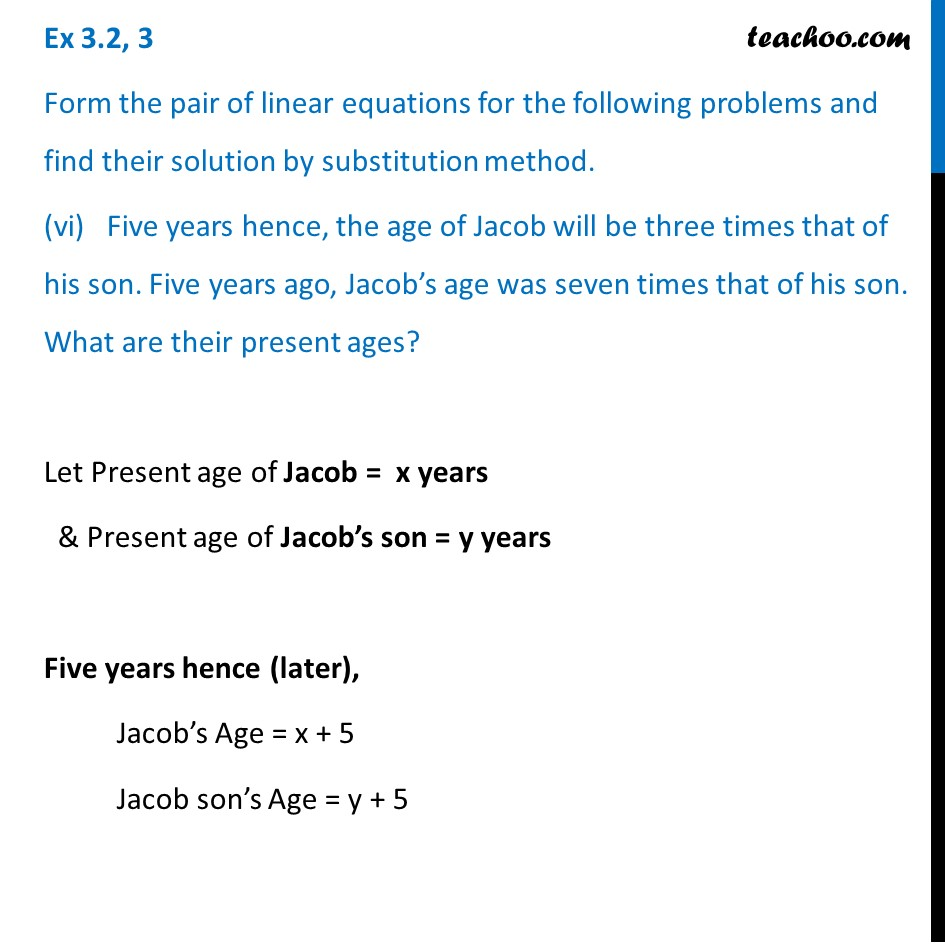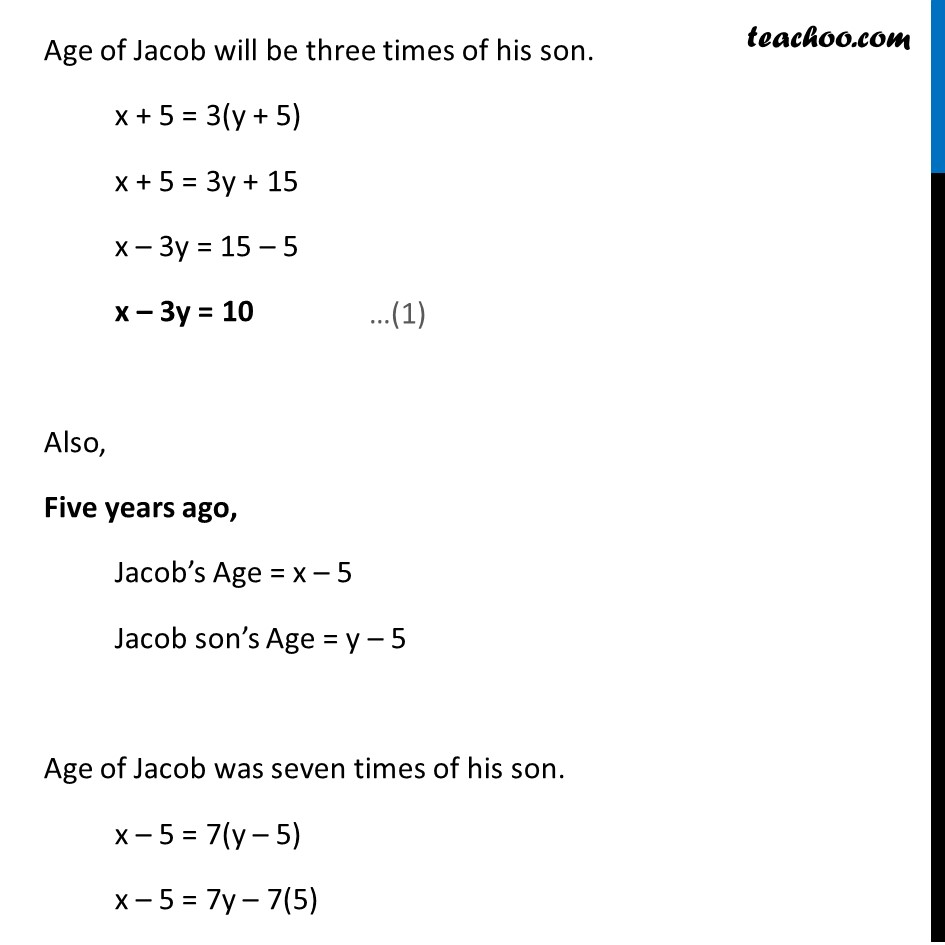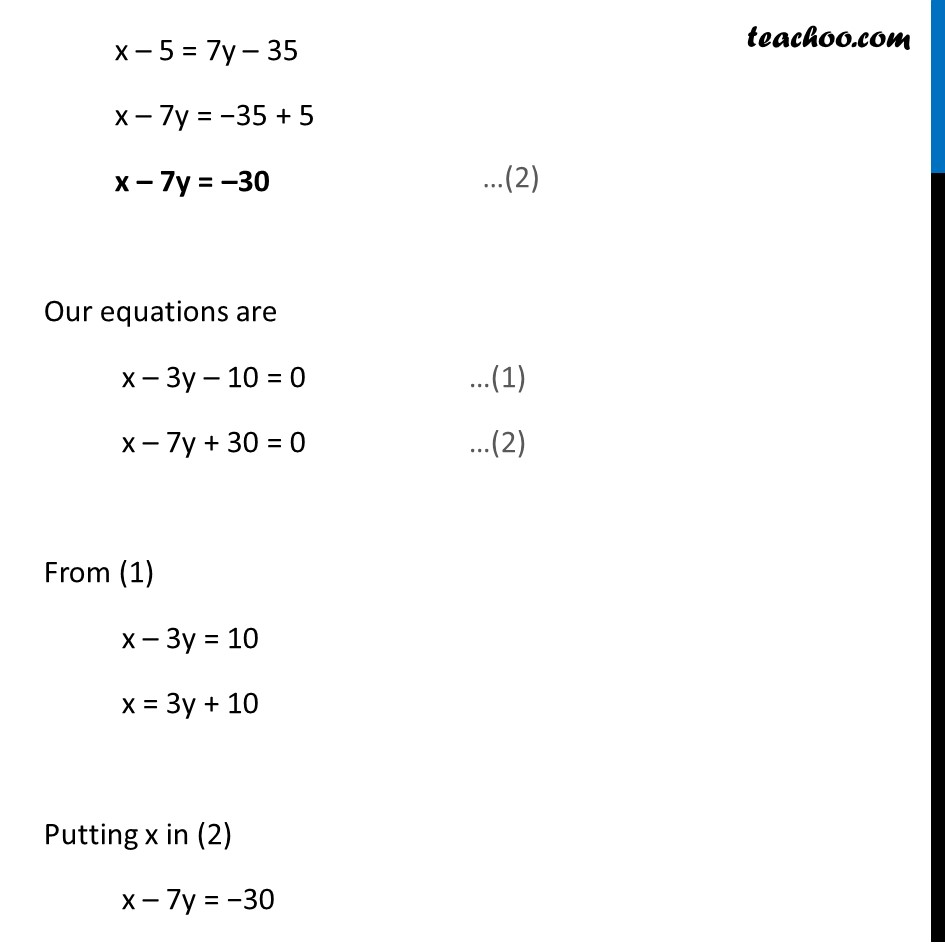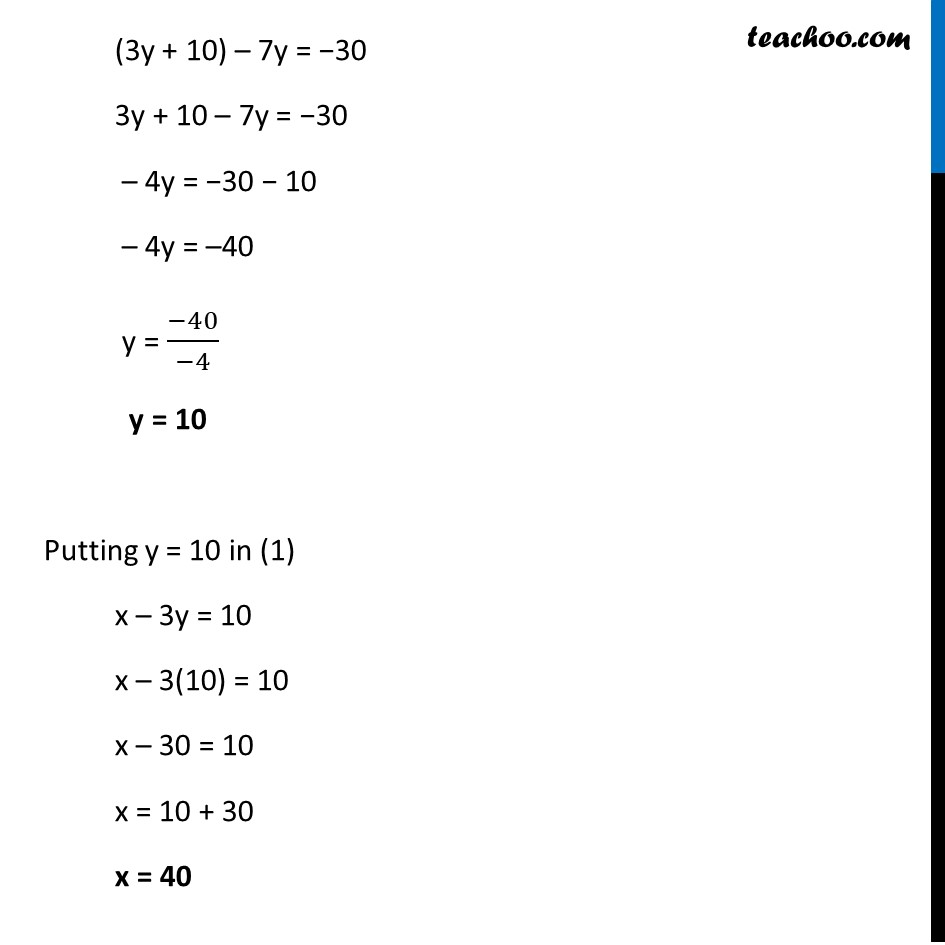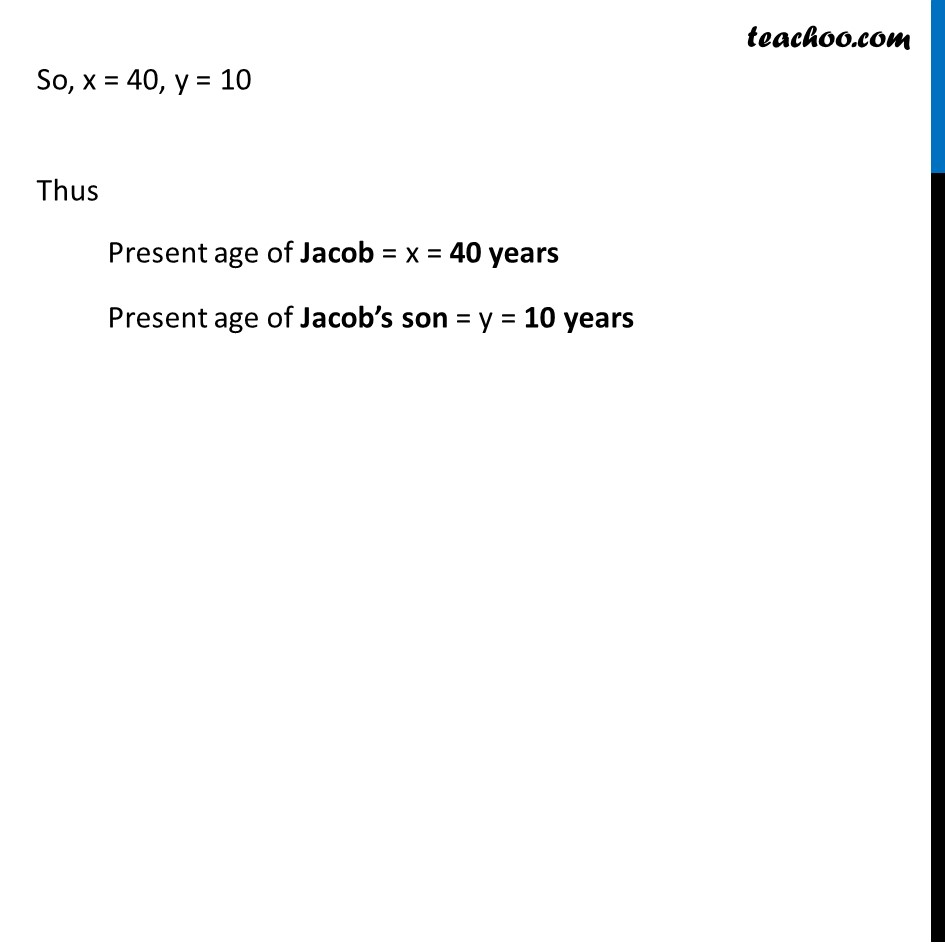Learn in your speed, with individual attention - Teachoo Maths 1-on-1 Class

### Transcript

Ex 3.2, 3 Form the pair of linear equations for the following problems and find their solution by substitution method. (vi) Five years hence, the age of Jacob will be three times that of his son. Five years ago, Jacob’s age was seven times that of his son. What are their present ages? Let Present age of Jacob = x years & Present age of Jacob’s son = y years Five years hence (later), Jacob’s Age = x + 5 Jacob son’s Age = y + 5 Age of Jacob will be three times of his son. x + 5 = 3(y + 5) x + 5 = 3y + 15 x – 3y = 15 – 5 x – 3y = 10 Also, Five years ago, Jacob’s Age = x – 5 Jacob son’s Age = y – 5 Age of Jacob was seven times of his son. x – 5 = 7(y – 5) x – 5 = 7y – 7(5) x – 5 = 7y – 35 x – 7y = −35 + 5 x – 7y = –30 Our equations are x – 3y – 10 = 0 …(1) x – 7y + 30 = 0 …(2) From (1) x – 3y = 10 x = 3y + 10 Putting x in (2) x – 7y = −30 (3y + 10) – 7y = −30 3y + 10 – 7y = −30 – 4y = −30 − 10 – 4y = –40 y = (−40)/(−4) y = 10 Putting y = 10 in (1) x – 3y = 10 x – 3(10) = 10 x – 30 = 10 x = 10 + 30 x = 40 So, x = 40, y = 10 Thus Present age of Jacob = x = 40 years Present age of Jacob’s son = y = 10 years